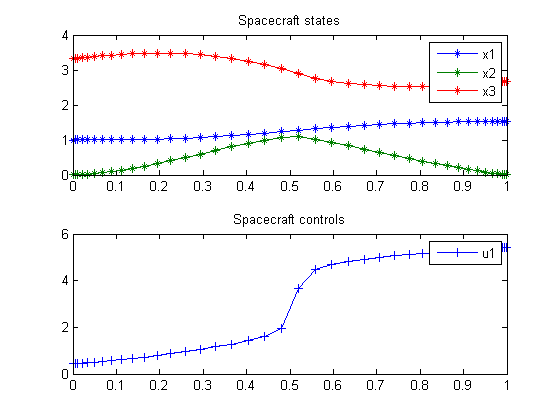63.1  Problem description

Maximum radius orbit transfer of a spacecraft.

Applied Optimal Control, Bryson & Ho, 1975. Example on pages 66-69.

Programmers: Gerard Van Willigenburg (Wageningen University) Willem De Koning (retired from Delft University of Technology)

63.2  Problem setup

% Array with consecutive number of collocation points
narr = [20 40];

toms t;
t_f = 1; % Fixed final time

for n=narr
p = tomPhase('p', t, 0, t_f, n);
setPhase(p)

tomStates x1 x2 x3
tomControls u1

% Parameters
r0 = 1; mmu = 11; th = 1.55;
m0 = 1; rm0 = -0.25;

% Initial state
xi=[r0; 0; sqrt(mmu/r0)];

% Initial guess
if n==narr(1)
x0 = {icollocate({x1 == xi(1); x2 == xi(2); x3 == xi(3)})
collocate({u1 == 0})};
else
x0 = {icollocate({x1 == xopt1; x2 == xopt2; x3 == xopt3})
collocate({u1 == uopt1})};
end

% Boundary constraints
cbnd = {initial({x1 == xi(1); x2 == xi(2); x3 == xi(3)})
final({x3 == sqrt(mmu/x1); x2 == 0})};

% ODEs and path constraints
dx1 = x2;
dx2 = x3.*x3./x1-mmu./(x1.*x1)+th*sin(u1)./(m0+rm0*t);
dx3 = -x2.*x3./x1+th*cos(u1)./(m0+rm0*t);

ceq = collocate({
dot(x1) == dx1
dot(x2) == dx2
dot(x3) == dx3});

% Objective
objective = -final(x1);

63.3  Solve the problem

options = struct;
options.name = 'Spacecraft';
solution = ezsolve(objective, {cbnd, ceq}, x0, options);

xopt1 = subs(x1,solution);
xopt2 = subs(x2,solution);
xopt3 = subs(x3,solution);
uopt1 = subs(u1,solution);
Problem type appears to be: lpcon
Starting numeric solver
===== * * * =================================================================== * * *
TOMLAB - Tomlab Optimization Inc. Development license  999001. Valid to 2011-02-05
=====================================================================================
Problem: ---  1: Spacecraft                     f_k      -1.526286382793847300
sum(|constr|)      0.000000153662086173
f(x_k) + sum(|constr|)     -1.526286229131761200
f(x_0)     -0.999999999999998220

Solver: snopt.  EXIT=0.  INFORM=1.
SNOPT 7.2-5 NLP code
Optimality conditions satisfied

FuncEv    1 ConstrEv   75 ConJacEv   75 Iter   43 MinorIter   96
CPU time: 0.234375 sec. Elapsed time: 0.235000 sec.
Problem type appears to be: lpcon
Starting numeric solver
===== * * * =================================================================== * * *
TOMLAB - Tomlab Optimization Inc. Development license  999001. Valid to 2011-02-05
=====================================================================================
Problem: ---  1: Spacecraft                     f_k      -1.526020732841172800
sum(|constr|)      0.000002928422557971
f(x_k) + sum(|constr|)     -1.526017804418614800
f(x_0)     -1.526286382793838200

Solver: snopt.  EXIT=0.  INFORM=1.
SNOPT 7.2-5 NLP code
Optimality conditions satisfied

FuncEv    1 ConstrEv   15 ConJacEv   15 Iter   13 MinorIter  157
CPU time: 0.171875 sec. Elapsed time: 0.172000 sec.
end

% Get final solution
t  = subs(collocate(t),solution);
x1 = subs(collocate(x1),solution);
x2 = subs(collocate(x2),solution);
x3 = subs(collocate(x3),solution);
u1 = subs(collocate(u1),solution);

%Bound u1 to [0,2pi]
u1 = rem(u1,2*pi); u1 = (u1<0)*2*pi+u1;

% Plot final solution
subplot(2,1,1)
plot(t,x1,'*-',t,x2,'*-',t,x3,'*-');
legend('x1','x2','x3');
title('Spacecraft states');

subplot(2,1,2)
plot(t,u1,'+-');
legend('u1');
title('Spacecraft controls');